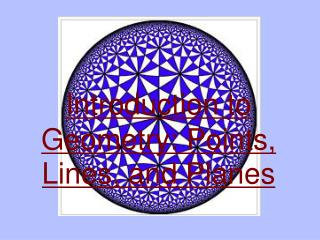DownloadDownload PresentationIntroduction to Geometry: Points, Lines, and Planes

# Introduction to Geometry: Points, Lines, and Planes

Download Presentation## Introduction to Geometry: Points, Lines, and Planes

- - - - - - - - - - - - - - - - - - - - - - - - - - - E N D - - - - - - - - - - - - - - - - - - - - - - - - - - -
##### Presentation Transcript

1. Introduction to Geometry: Points, Lines, and Planes

2. Introduction to Geometry • This course will be much different from any other math class you have had before, it is much less about numbers and more about words. • Geometry will be like learning a new language. • Lots of new vocabulary terms, symbols, and rules.

3. The Rules • Postulates - statements that are accepted without any proof. • Axioms - statements accepted without proof necessary - usually related to a postulate. • Theorems - statements that can be proven using definitions, postulates, and axioms. • Corollaries - an additional part of a theorem.

4. Essential Vocabulary These are terms you must be most familiar with, as they will be an integral part of the course.

5. P Point Written as: P No size or dimension, merely position. Written using a single, capital letter. Points are equidistant, if they are the same measurable distance away from a given location.

6. h A B C Written as: Line Contains infinitely many points extending in opposite directions. Any two points name the line, order does NOT matter.

7. M Plane Written as:M An imaginary, perfectly flat surface with no thickness that extends endlessly in all directions. Plane can be named using a single capital letter OR using three or four points. Ex. Plane M or Plane ABC or ABC

8. A B C Collinear Points Points that lie on the same line. A, B, and C are collinear points.

9. R P Q S Noncollinear Points Ex. P and R are noncollinear points. Ex. S and P are noncollinear points. Points that do not lie on the same line.

10. P M N Coplanar Points Points that lie on the same plane. P, M, and N are coplanar points.

11. B C A E D N A B M Noncoplanar Points Points that do not lie on the same plane. A, B, M, and N are noncoplanar points.

12. R Intersection The set of points that lie in both figures. R is the intersection of the two lines.

13. F E B C D C E G D A A B Diagram Use the diagram to answer the following.

14. Questions… • Are A, D, C, and F are coplanar ? • Are D, E, B, and G are coplanar ? • How many points are contained in Plane ABCD ? • Can a point be in more than one line ? • Name the intersection of Plane DEFC and Plane BGFC ? • Name two planes that do not intersect.